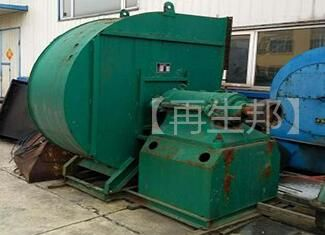•• 40t锅炉配套鼓引风机3台： 离心式通风机，Q=61200m³/h，F=3400Pa； 三相异步电动机，N=90KW； 低噪声通风机，Q=50533m³/h，F=3400Pa； 三相异步电动机，N=55KW； 低噪声引风机，Q=186100m³/h，F=2676Pa； 三相异步电动机，N=185KW。 离心式通风机2台： 离心式通风机（小），Q=3600m³/h，F=2200Pa； 三相异步电动机，N=3KW； 离心式通风机（小），Q=1810m³/h，F=843Pa； 三相异步电动机，N=1.5KW。 锅炉配套减速机，速比40.17； 供热用循环水泵4台： 单级离心泵，DFG200-400(1)/4，Q=280m³/h，H=54.5m，2台； 单级离心泵，DFG200-315(1)/4，Q=400m³/h，H=32m，2台。 供水用离心泵12台： QDL系列立式多级离心泵，Q=2.4m³/h，H=144m，N=3KW； 清水离心式水泵，Q=10m³/h H=36m，N=3KW； LG型立式高层给水泵，Q=5.6m³/h，H=16m，N=2.2KW； 单级离心泵，Q=4.1m³/h，H=72m， N=5.5KW，4台； 单级离心泵，Q=2.0m³/h，H=60m，N=7.5KW，4台。

•